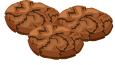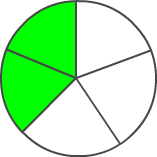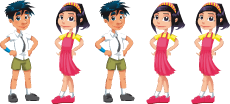# What is Fraction?

Rate Lesson 0 stars
Lesson size:
Message preview:
Someone you know has shared lesson with you:

To play this lesson, click on the link below:

https://www.turtlediary.com/lesson/what-is-fraction.html

Hope you have a good experience with this site and recommend to your friends too.

Login to rate activities and track progress.
Login to rate activities and track progress.

If four kids are sharing 8 cookies equally, each kid gets two cookies.

But what if four friends are sharing 3 cookies equally?

How much cookie does each kid get?To represent parts of whole numbers, we use fractions.

So if 4 kids are sharing 3 cookies, what happens?

First, we divide one cookie into four equal parts, and give one part to each kid. Then we do the same with the second cookie, and then with the third one.

In the end, each kid will have three pieces. Each piece is a whole cookie divided into four equal parts.

So we represent this amount as

3 (Number of parts) & 4 (How many equal parts the whole is divided into)

3
4
as a fraction

A fraction is made of two numbers :

## Numerator

The numerator is the number on the top, and shows you how many parts of the whole you have.

## Denominator

The bottom number is called the denominator. It shows how many equal parts the whole is divided into.

### Example 1

The whole circle is divided into five equal pieces, and two of them are shaded. Represent the shaded part of the circle as a fraction.Two out of five pieces are shaded.

This fraction is
2
5
.

## Can fractions also be bigger than 1 ??

For example, if 3 children are sharing 8 sandwiches equally, each child will get
8
3
of a sandwich.
Think about how this happens - we divide the first sandwich into three equal parts and hand them out to the kids. We do the same with the second sandwich, the third sandwich, and so on. By the time we're done, each kid will have 8 pieces, each of which represents a sandwich cut into 3 equal parts. This is how we get
8
3
.

8
3
is bigger than one. Why?

Well, if each piece is one-third of a sandwich, three pieces together will be an entire sandwich! But the kids have 8 pieces. So that's one whole sandwich plus five more pieces. In fact, it's equal to two whole sandwiches plus 2 more pieces.

### Remember !!! Each sandwich was cut into 3 equal parts.

So, the remaining 2 pieces can be written as fraction:
2
3

Here's an easy way to tell if a fraction is larger than 1 or not:

If the numerator is bigger than the denominator, the fraction is bigger than 1.

Let's look at some more fractions:

### Example 2

Write a fraction that tells how many of the cars are blue.1 of the 5 cars are blue. We can write this as this fraction:
1
5

1 is the numerator because it tells how many cars are blue, and 5 is the denominator because it tells how many total cars there are.

### Example 3

Write a fraction that tells how many of the keys are yellow.2 of the 3 cars are yellow. We can write this as this fraction:
2
3

2 is the numerator because it tells how many keys are yellow, and 3 is the denominator because it tells how many total keys there are.

### Example 4

In Olivia's family, there are 2 boys and 3 girls. Write a fraction that tells how many of the children are boys.First we have to figure out how many total children there are

2 + 3 = 5 , so there are 5 kids total.

That means 2 out of 5 children are boys.

We can write that as a fraction:
2
5

2 is the numerator because it tells how many of the children are boys, and 5 is the denominator because it tells how many total children there are.

Summary
• Fractions represent parts of whole numbers
• Numerator (number on top) represents how many parts of the whole you have
• Denominator (number on the bottom) represents how many equal parts the whole is divided into.

## More Fractions Games

Become premium member to get unlimited access.